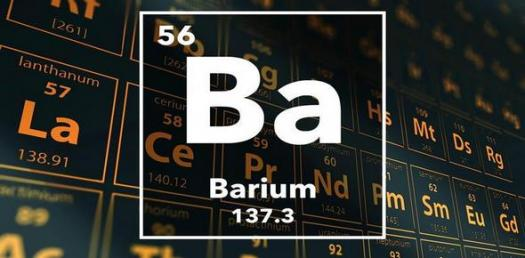10 Questions | Total Attempts: 114SettingsBarium is a chemical element which is symbolized with Ba and which is also a member of group 2. It is a soft, silvery alkaline earth metal and because of its high chemical reactivity, it is never found as a free element in nature. The most common naturally occurring minerals of Barium are barite. This short quiz is to put your knowledge of Barium to test to ascertain all that you may know as well as those you don't.

• 1.
Which of the following is the atomic number of Barium?
• A.

39

• B.

48

• C.

56

• D.

69

• 2.
What position is Barium in group 2?
• A.

Fifth

• B.

First

• C.

Third

• D.

Second

• 3.
Which of the following is the Pauling scale of Barium?
• A.

0.89

• B.

7.12

• C.

1.53

• D.

0.95

• 4.
Which of the following is the Bulk modulus of Barium?
• A.

10.16 GPa

• B.

7.15 GPa

• C.

9.57 GPa

• D.

9.6 GPa

• 5.
What is the Shear modulus of Barium?
• A.

4.9 GPa

• B.

5.9 GPa

• C.

8.5 GPa

• D.

3.5 GPa

• 6.
Which of the following is the Mohs hardness of Barium?
• A.

1.25

• B.

18.4

• C.

2.72

• D.

6.59

• 7.
When was Barium identified as an element?
• A.

1887

• B.

1774

• C.

1508

• D.

1769

• 8.
When was Barium reduced to a metal?
• A.

1808

• B.

1758

• C.

1793

• D.

1825

• 9.
When Barium compounds are added to fireworks, which colour is the outcome?
• A.

Red

• B.

Yellow

• C.

Blue

• D.

Green

• 10.
Which of the following is true about barium ion and soluble compounds?
• A.

They are good for fireworks

• B.

They're used for making salt

• C.

They're good ingredients for air fresheners

• D.

They're poisonous

Related TopicsBack to top